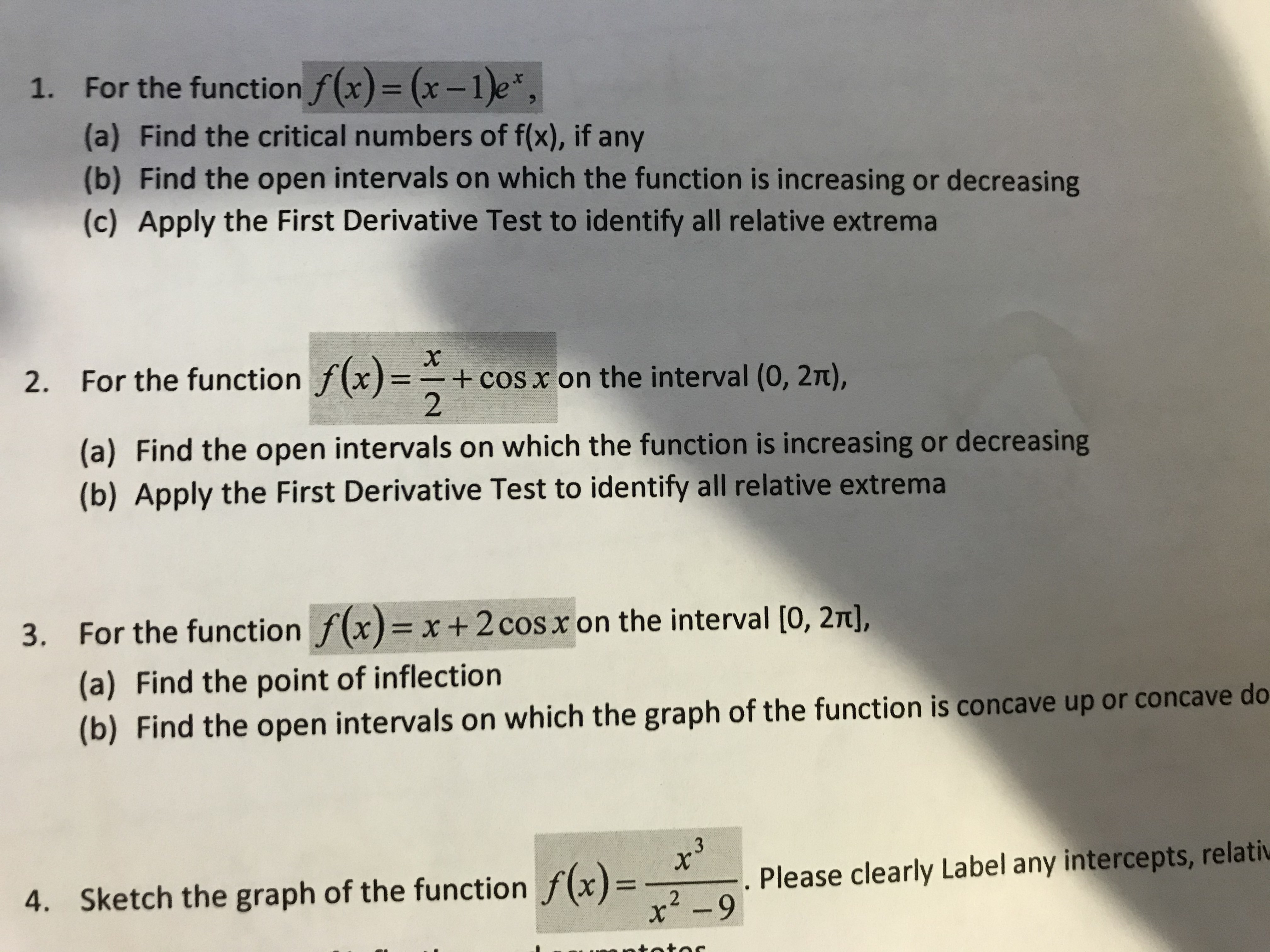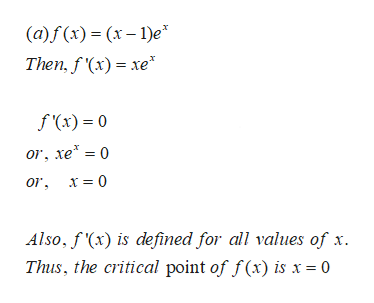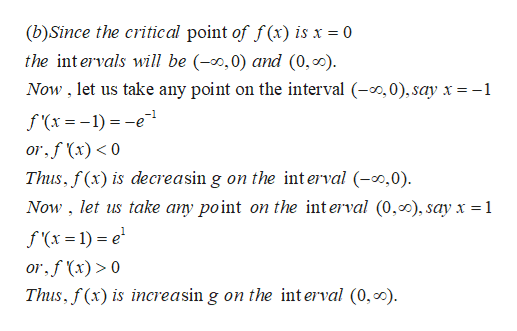# 1.For the function f(x) = (x-1)e,(a) Find the critical numbers of f(x), if any(b) Find the open intervals on which the function is increasing or decreasing(c) Apply the First Derivative Test to identify all relative extrema2. For the function f(x)=+ cos x on the interval (0, 2t),2(a) Find the open intervals on which the function is increasing or decreasing(b) Apply the First Derivative Test to identify all relative extrema3. For the function f(x) x+ 2 cos x on the interval [0, 2T],(a) Find the point of inflection(b) Find the open intervals on which the graph of the function is concave up or concave do3Sketch the graph of the function f(x)Please clearly Label any intercepts, relativ4.x2-9

Question
3 views

numberr 2

full workshelp_outlineImage Transcriptionclose1. For the function f(x) = (x-1)e, (a) Find the critical numbers of f(x), if any (b) Find the open intervals on which the function is increasing or decreasing (c) Apply the First Derivative Test to identify all relative extrema 2. For the function f(x)= + cos x on the interval (0, 2t), 2 (a) Find the open intervals on which the function is increasing or decreasing (b) Apply the First Derivative Test to identify all relative extrema 3. For the function f(x) x+ 2 cos x on the interval [0, 2T], (a) Find the point of inflection (b) Find the open intervals on which the graph of the function is concave up or concave do 3 Sketch the graph of the function f(x) Please clearly Label any intercepts, relativ 4. x2-9 fullscreen
check_circle

Step 1

Hi there !!! Thank you for the question. But there are multiple questions in this request. So, we will be answering the first question. In case you need the solution of the other questions, please ask them in a new request, specifying the question number.

(a) Let us find where f'(x) is zero and undefined, and then the critical point:help_outlineImage Transcriptionclose(a)f (x) x-1)e Then, fx)xe f (x) = 0 or, xe 0 or x= 0 Also, f'(x) is defined for all values of x Thus, the critical point of f(x) is x = 0 fullscreen
Step 2

(b) Increasing and decre...help_outlineImage Transcriptionclose(b)Since the critical point of f(x) is x = 0 the int ervals will be (-,0) and (0,0) Now, let us take any point on the interval (-,0), say x -1 f (x1)-e or,f(x)0 Thus, f(x) is decreasin g on the interval (0) Now, let us take any point on the interval (0,0), say x = 1 f (x=1)e or,f (x)> 0 Thus, f(x) is increasin g on the int erval (0,0). fullscreen

### Want to see the full answer?

See Solution

#### Want to see this answer and more?

Solutions are written by subject experts who are available 24/7. Questions are typically answered within 1 hour.*

See Solution
*Response times may vary by subject and question.
Tagged in

### Other